3.1 Functions and function notation  (Page 10/21)

 Page 10 / 21

Why does the horizontal line test tell us whether the graph of a function is one-to-one?

When a horizontal line intersects the graph of a function more than once, that indicates that for that output there is more than one input. A function is one-to-one if each output corresponds to only one input.

Algebraic

For the following exercises, determine whether the relation represents a function.

$\left\{\left(a,b\right),\left(b,c\right),\left(c,c\right)\right\}$

function

For the following exercises, determine whether the relation represents $\text{\hspace{0.17em}}y\text{\hspace{0.17em}}$ as a function of $\text{\hspace{0.17em}}x.\text{\hspace{0.17em}}$

$5x+2y=10$

$y={x}^{2}$

function

$x={y}^{2}$

$3{x}^{2}+y=14$

function

$2x+{y}^{2}=6$

$y=-2{x}^{2}+40x$

function

$y=\frac{1}{x}$

$x=\frac{3y+5}{7y-1}$

function

$x=\sqrt{1-{y}^{2}}$

$y=\frac{3x+5}{7x-1}$

function

${x}^{2}+{y}^{2}=9$

$2xy=1$

function

$x={y}^{3}$

$y={x}^{3}$

function

$y=\sqrt{1-{x}^{2}}$

$x=±\sqrt{1-y}$

function

$y=±\sqrt{1-x}$

${y}^{2}={x}^{2}$

not a function

${y}^{3}={x}^{2}$

For the following exercises, evaluate the function $\text{\hspace{0.17em}}f\text{\hspace{0.17em}}$ at the indicated values

$f\left(x\right)=2x-5$

$\begin{array}{cccc}f\left(-3\right)=-11;& f\left(2\right)=-1;& f\left(-a\right)=-2a-5;& -f\left(a\right)=-2a+5;\text{\hspace{0.17em}}\text{\hspace{0.17em}}\text{\hspace{0.17em}}\text{\hspace{0.17em}}f\left(a+h\right)=2a+2h-5\end{array}$

$f\left(x\right)=-5{x}^{2}+2x-1$

$f\left(x\right)=\sqrt{2-x}+5$

$\begin{array}{cccc}f\left(-3\right)=\sqrt{5}+5;& f\left(2\right)=5;& f\left(-a\right)=\sqrt{2+a}+5;& -f\left(a\right)=-\sqrt{2-a}-5;\text{\hspace{0.17em}}\text{\hspace{0.17em}}\text{\hspace{0.17em}}\text{\hspace{0.17em}}\text{\hspace{0.17em}}f\left(a+h\right)=\end{array}$ $\sqrt{2-a-h}+5$

$f\left(x\right)=\frac{6x-1}{5x+2}$

$f\left(x\right)=|x-1|-|x+1|$

Given the function $\text{\hspace{0.17em}}g\left(x\right)=5-{x}^{2},\text{\hspace{0.17em}}$ simplify $\text{\hspace{0.17em}}\frac{g\left(x+h\right)-g\left(x\right)}{h},\text{\hspace{0.17em}}h\ne 0.$

Given the function $\text{\hspace{0.17em}}g\left(x\right)={x}^{2}+2x,\text{\hspace{0.17em}}$ simplify $\text{\hspace{0.17em}}\frac{g\left(x\right)-g\left(a\right)}{x-a},\text{\hspace{0.17em}}x\ne a.$

$\frac{g\left(x\right)-g\left(a\right)}{x-a}=x+a+2,\text{\hspace{0.17em}}x\ne a$

Given the function $\text{\hspace{0.17em}}k\left(t\right)=2t-1\text{:}$

1. Evaluate $\text{\hspace{0.17em}}k\left(2\right).$
2. Solve $\text{\hspace{0.17em}}k\left(t\right)=7.$

Given the function $\text{\hspace{0.17em}}f\left(x\right)=8-3x\text{:}$

1. Evaluate $\text{\hspace{0.17em}}f\left(-2\right).$
2. Solve $\text{\hspace{0.17em}}f\left(x\right)=-1.$

a. $\text{\hspace{0.17em}}f\left(-2\right)=14;\text{\hspace{0.17em}}$ b. $\text{\hspace{0.17em}}x=3$

Given the function $\text{\hspace{0.17em}}p\left(c\right)={c}^{2}+c\text{:}$

1. Evaluate $\text{\hspace{0.17em}}p\left(-3\right).$
2. Solve $\text{\hspace{0.17em}}p\left(c\right)=2.$

Given the function $\text{\hspace{0.17em}}f\left(x\right)={x}^{2}-3x\text{:}$

1. Evaluate $\text{\hspace{0.17em}}f\left(5\right).$
2. Solve $\text{\hspace{0.17em}}f\left(x\right)=4.$

a. $\text{\hspace{0.17em}}f\left(5\right)=10;\text{\hspace{0.17em}}$ b. or

Given the function $\text{\hspace{0.17em}}f\left(x\right)=\sqrt{x+2}\text{:}$

1. Evaluate $\text{\hspace{0.17em}}f\left(7\right).$
2. Solve $\text{\hspace{0.17em}}f\left(x\right)=4.$

Consider the relationship $\text{\hspace{0.17em}}3r+2t=18.$

1. Write the relationship as a function $\text{\hspace{0.17em}}r=f\left(t\right).$
2. Evaluate $\text{\hspace{0.17em}}f\left(-3\right).$
3. Solve $\text{\hspace{0.17em}}f\left(t\right)=2.$

a. $\text{\hspace{0.17em}}f\left(t\right)=6-\frac{2}{3}t;\text{\hspace{0.17em}}$ b. $\text{\hspace{0.17em}}f\left(-3\right)=8;\text{\hspace{0.17em}}$ c. $\text{\hspace{0.17em}}t=6\text{\hspace{0.17em}}$

Graphical

For the following exercises, use the vertical line test to determine which graphs show relations that are functions.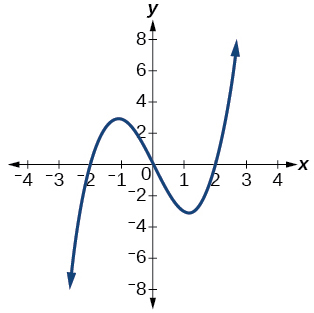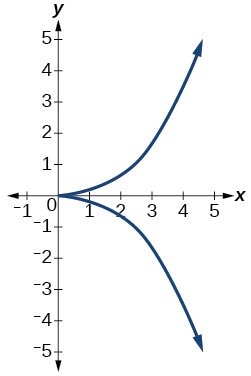not a function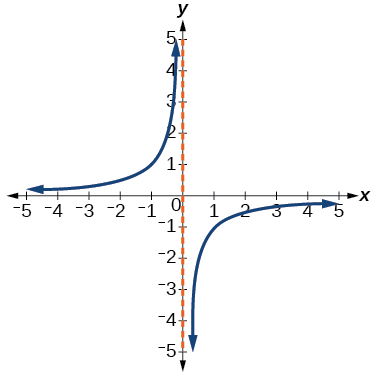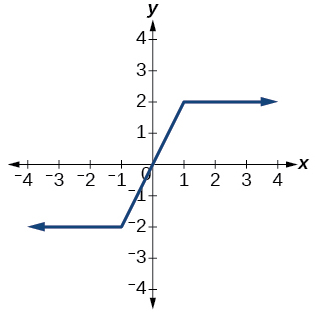function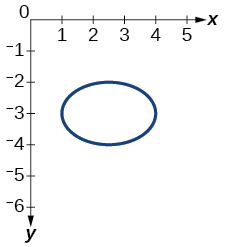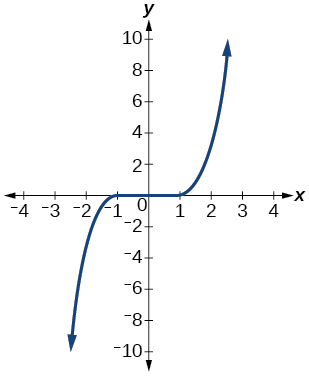function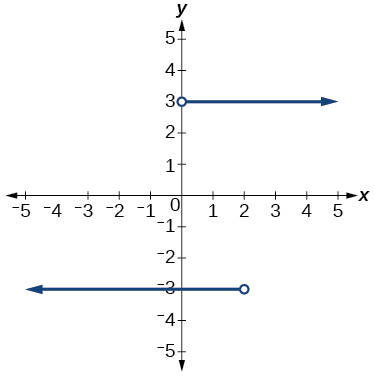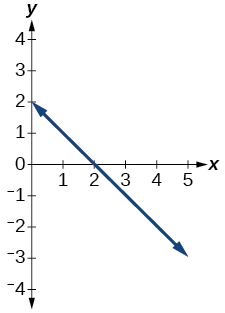function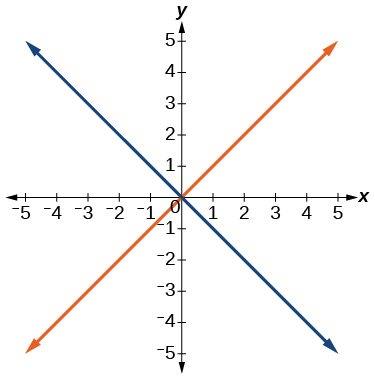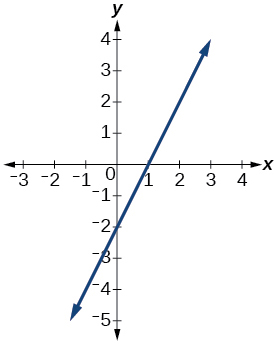function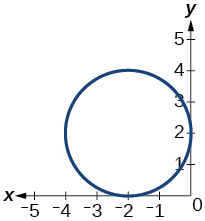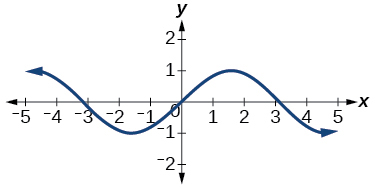function

Given the following graph,

• Evaluate $\text{\hspace{0.17em}}f\left(-1\right).$
• Solve for $\text{\hspace{0.17em}}f\left(x\right)=3.$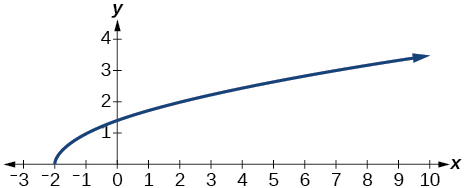Given the following graph,

• Evaluate $\text{\hspace{0.17em}}f\left(0\right).$
• Solve for $\text{\hspace{0.17em}}f\left(x\right)=-3.$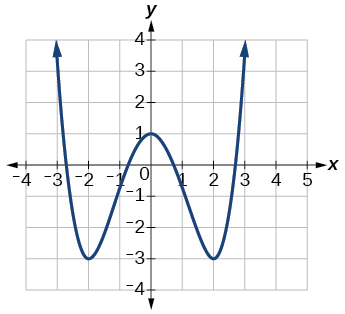a. $\text{\hspace{0.17em}}f\left(0\right)=1;\text{\hspace{0.17em}}$ b. or

Given the following graph,

• Evaluate $\text{\hspace{0.17em}}f\left(4\right).$
• Solve for $\text{\hspace{0.17em}}f\left(x\right)=1.$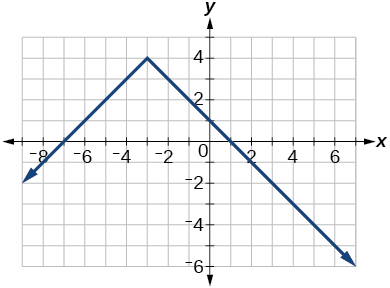For the following exercises, determine if the given graph is a one-to-one function.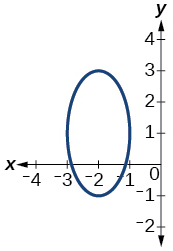not a function so it is also not a one-to-one function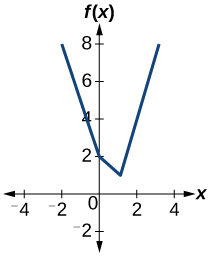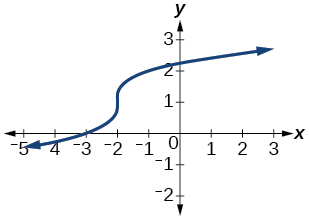one-to- one function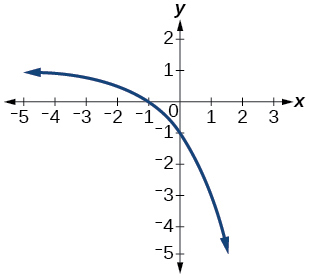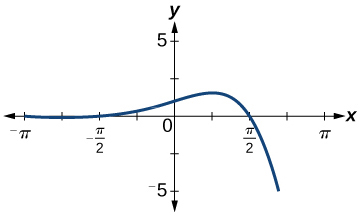function, but not one-to-one

Numeric

For the following exercises, determine whether the relation represents a function.

$\left\{\left(-1,-1\right),\left(-2,-2\right),\left(-3,-3\right)\right\}$

$\left\{\left(3,4\right),\left(4,5\right),\left(5,6\right)\right\}$

function

$\left\{\left(2,5\right),\left(7,11\right),\left(15,8\right),\left(7,9\right)\right\}$

For the following exercises, determine if the relation represented in table form represents $\text{\hspace{0.17em}}y\text{\hspace{0.17em}}$ as a function of $\text{\hspace{0.17em}}x.$

 $x$ 5 10 15 $y$ 3 8 14

function

 $x$ 5 10 15 $y$ 3 8 8
 $x$ 5 10 10 $y$ 3 8 14

not a function

For the following exercises, use the function $\text{\hspace{0.17em}}f\text{\hspace{0.17em}}$ represented in [link] .

 $x$ $f\left(x\right)$ 0 74 1 28 2 1 3 53 4 56 5 3 6 36 7 45 8 14 9 47

Evaluate $\text{\hspace{0.17em}}f\left(3\right).$

Solve $\text{\hspace{0.17em}}f\left(x\right)=1.$

$f\left(x\right)=1,\text{\hspace{0.17em}}x=2$

For the following exercises, evaluate the function $\text{\hspace{0.17em}}f\text{\hspace{0.17em}}$ at the values $f\left(-2\right),\text{\hspace{0.17em}}f\left(-1\right),\text{\hspace{0.17em}}f\left(0\right),\text{\hspace{0.17em}}f\left(1\right),$ and $\text{\hspace{0.17em}}f\left(2\right).$

$f\left(x\right)=4-2x$

$f\left(x\right)=8-3x$

$\begin{array}{ccccc}f\left(-2\right)=14;& f\left(-1\right)=11;& f\left(0\right)=8;& f\left(1\right)=5;& f\left(2\right)=2\end{array}$

$f\left(x\right)=8{x}^{2}-7x+3$

$f\left(x\right)=3+\sqrt{x+3}$

$\begin{array}{ccccc}f\left(-2\right)=4;\text{ }& f\left(-1\right)=4.414;& f\left(0\right)=4.732;& f\left(1\right)=4.5;& f\left(2\right)=5.236\end{array}$

$f\left(x\right)=\frac{x-2}{x+3}$

$f\left(x\right)={3}^{x}$

$\begin{array}{ccccc}f\left(-2\right)=\frac{1}{9};& f\left(-1\right)=\frac{1}{3};& f\left(0\right)=1;& f\left(1\right)=3;& f\left(2\right)=9\end{array}$

For the following exercises, evaluate the expressions, given functions $f,\text{\hspace{0.17em}}\text{\hspace{0.17em}}g,$ and $\text{\hspace{0.17em}}h\text{:}$

• $f\left(x\right)=3x-2$
• $g\left(x\right)=5-{x}^{2}$
• $h\left(x\right)=-2{x}^{2}+3x-1$

$3f\left(1\right)-4g\left(-2\right)$

$f\left(\frac{7}{3}\right)-h\left(-2\right)$

20

Technology

For the following exercises, graph $\text{\hspace{0.17em}}y={x}^{2}\text{\hspace{0.17em}}$ on the given viewing window. Determine the corresponding range for each viewing window. Show each graph.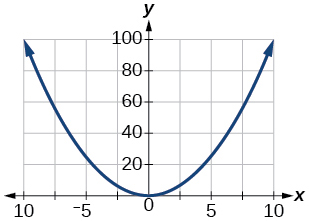$\left[-100,100\right]$

For the following exercises, graph $\text{\hspace{0.17em}}y={x}^{3}\text{\hspace{0.17em}}$ on the given viewing window. Determine the corresponding range for each viewing window. Show each graph.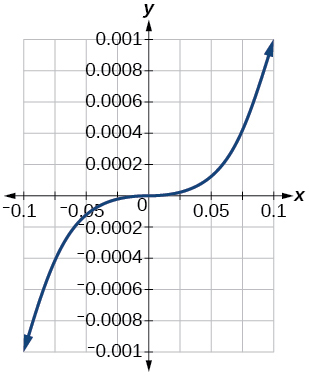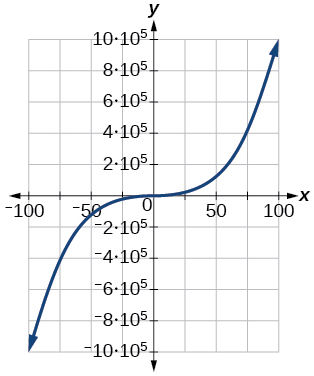For the following exercises, graph $\text{\hspace{0.17em}}y=\sqrt{x}\text{\hspace{0.17em}}$ on the given viewing window. Determine the corresponding range for each viewing window. Show each graph.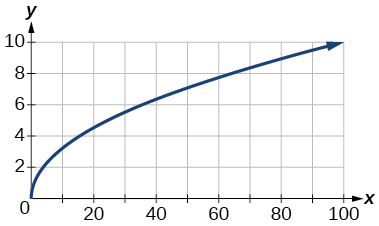For the following exercises, graph $y=\sqrt{x}$ on the given viewing window. Determine the corresponding range for each viewing window. Show each graph.

$\left[-0.001,\text{0.001}\right]$

$\left[-0.1,\text{0.1}\right]$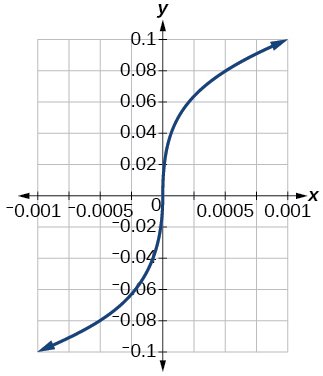$\left[-1000,\text{1000}\right]$

$\left[-1,000,000,\text{1,000,000}\right]$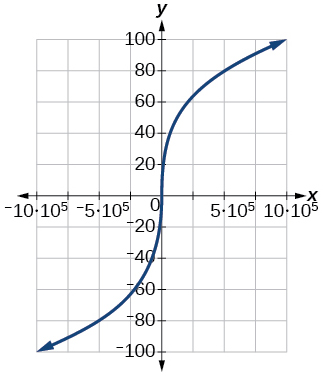Real-world applications

The amount of garbage, $\text{\hspace{0.17em}}G,\text{\hspace{0.17em}}$ produced by a city with population $\text{\hspace{0.17em}}p\text{\hspace{0.17em}}$ is given by $\text{\hspace{0.17em}}G=f\left(p\right).\text{\hspace{0.17em}}$ $G\text{\hspace{0.17em}}$ is measured in tons per week, and $\text{\hspace{0.17em}}p\text{\hspace{0.17em}}$ is measured in thousands of people.

1. The town of Tola has a population of 40,000 and produces 13 tons of garbage each week. Express this information in terms of the function $\text{\hspace{0.17em}}f.\text{\hspace{0.17em}}$
2. Explain the meaning of the statement $\text{\hspace{0.17em}}f\left(5\right)=2.$

The number of cubic yards of dirt, $\text{\hspace{0.17em}}D,\text{\hspace{0.17em}}$ needed to cover a garden with area $\text{\hspace{0.17em}}a\text{\hspace{0.17em}}$ square feet is given by $\text{\hspace{0.17em}}D=g\left(a\right).$

1. A garden with area 5000 ft 2 requires 50 yd 3 of dirt. Express this information in terms of the function $\text{\hspace{0.17em}}g.$
2. Explain the meaning of the statement $\text{\hspace{0.17em}}g\left(100\right)=1.$

a. $\text{\hspace{0.17em}}g\left(5000\right)=50;$ b. The number of cubic yards of dirt required for a garden of 100 square feet is 1.

Let $\text{\hspace{0.17em}}f\left(t\right)\text{\hspace{0.17em}}$ be the number of ducks in a lake $\text{\hspace{0.17em}}t\text{\hspace{0.17em}}$ years after 1990. Explain the meaning of each statement:

1. $f\left(5\right)=30$
2. $f\left(10\right)=40$

Let $\text{\hspace{0.17em}}h\left(t\right)\text{\hspace{0.17em}}$ be the height above ground, in feet, of a rocket $\text{\hspace{0.17em}}t\text{\hspace{0.17em}}$ seconds after launching. Explain the meaning of each statement:

1. $h\left(1\right)=200$
2. $h\left(2\right)=350$

a. The height of a rocket above ground after 1 second is 200 ft. b. the height of a rocket above ground after 2 seconds is 350 ft.

Show that the function $\text{\hspace{0.17em}}f\left(x\right)=3{\left(x-5\right)}^{2}+7\text{\hspace{0.17em}}$ is not one-to-one.

The sequence is {1,-1,1-1.....} has
how can we solve this problem
Sin(A+B) = sinBcosA+cosBsinA
Prove it
Eseka
Eseka
hi
Joel
June needs 45 gallons of punch. 2 different coolers. Bigger cooler is 5 times as large as smaller cooler. How many gallons in each cooler?
7.5 and 37.5
Nando
find the sum of 28th term of the AP 3+10+17+---------
I think you should say "28 terms" instead of "28th term"
Vedant
the 28th term is 175
Nando
192
Kenneth
if sequence sn is a such that sn>0 for all n and lim sn=0than prove that lim (s1 s2............ sn) ke hole power n =n
write down the polynomial function with root 1/3,2,-3 with solution
if A and B are subspaces of V prove that (A+B)/B=A/(A-B)
write down the value of each of the following in surd form a)cos(-65°) b)sin(-180°)c)tan(225°)d)tan(135°)
Prove that (sinA/1-cosA - 1-cosA/sinA) (cosA/1-sinA - 1-sinA/cosA) = 4
what is the answer to dividing negative index
In a triangle ABC prove that. (b+c)cosA+(c+a)cosB+(a+b)cisC=a+b+c.
give me the waec 2019 questions
the polar co-ordinate of the point (-1, -1)ByByBy Lakeima RobertsByBy Dravida Mahadeo-J...